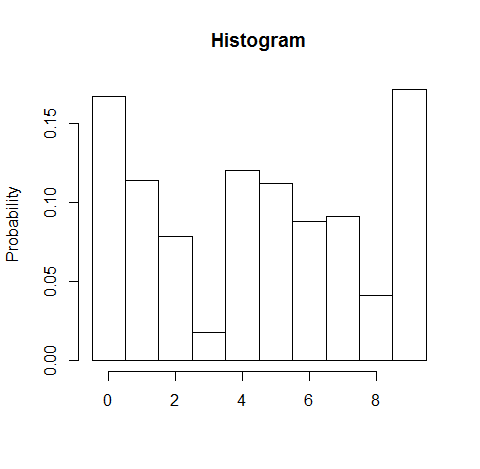# R: Create a histogram from frequency list

In R, `hist` is a great function to create histograms from data. It counts data, compute frequency, and show a histogram. Then how can I get histograms from frequency data that I already have? I wasn’t able to find nicer solution, so wrote this.

``> hist.from.freq(seq(0, 9), c(154, 105, 72, 16, 111, 103, 81, 84, 38, 158), normalize = T)``

This sample gives the following output: